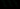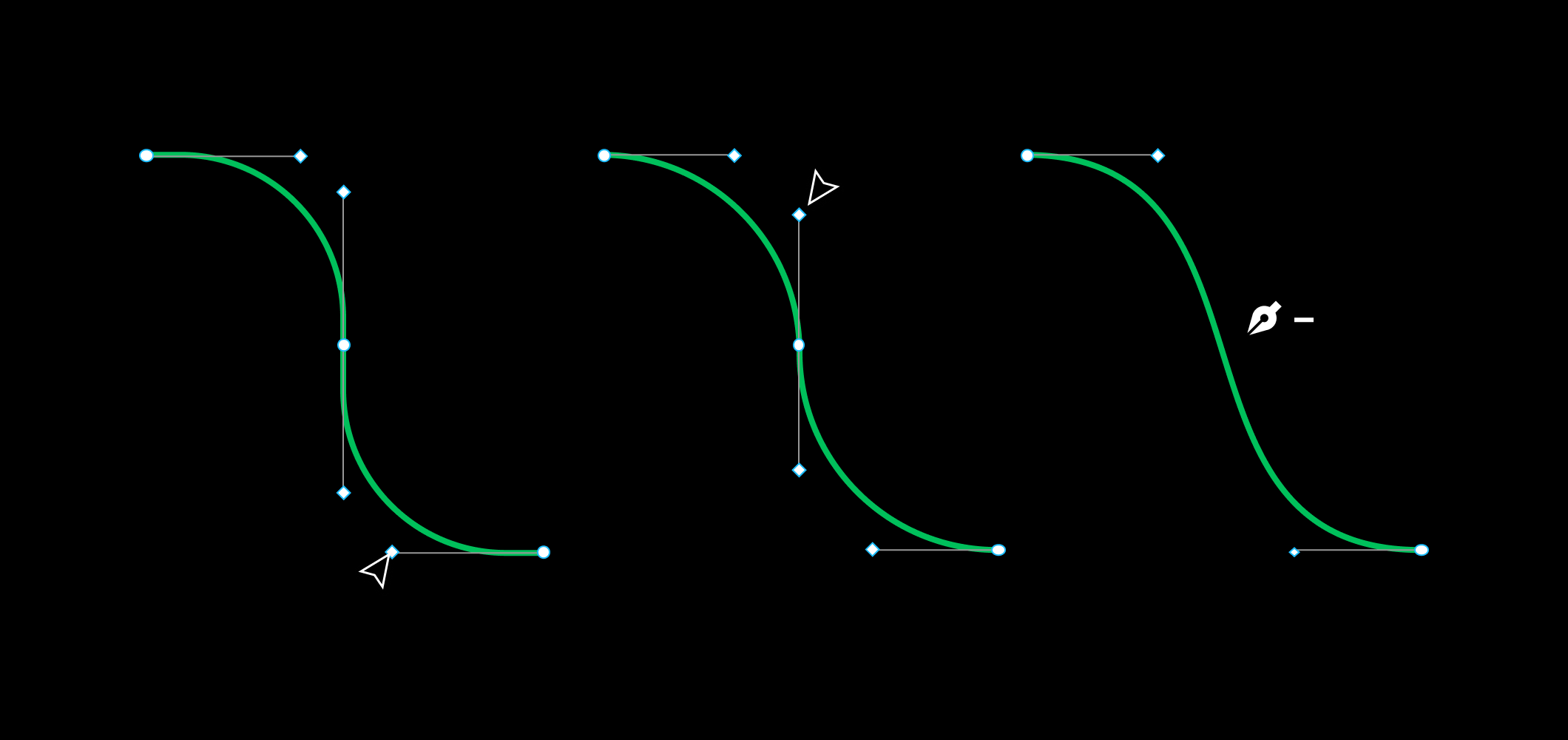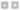# Delete and Heal for Vector Networks

JW
Jamie WongSince I joined Figma in September, among many other things, I had the pleasure of working on the intuitive yet surprisingly complex behavior you see above (You might need to give it a second to load the GIF).

Since I joined Figma in September, among many other things, I had the pleasure of working on the intuitive yet surprisingly complex behavior you see above (You might need to give it a second to load the GIF).

When implementing deletion of a vertex in a vector editing tool, the easiest thing to do is look at all the edges that touch that vertex and delete them too. If there are any fills touching those removed edges, throw those out too.

The distinction between “delete” and “delete and heal” is, as you might expect, the “heal” part. At first glance, this operation might seem like it has a straightforward implementation: join up the two vertices in the path that are adjacent to the deleted vertex.

Even in these simple examples, you start to run into edge cases. What happens if the vertex only touches one edge? In that case, you throw away the edge, since there’s no sensible “healing” action. What happens when you have a triangle and delete and heal one of the vertices? You have two vertices now, but should you be left with one edge or two? Figma’s answer is, well, it depends.

All the examples so far, while a little tricky, come mostly down to finding the edge cases and dealing with them sensibly. The problem gets really interesting once you consider how to deal with healing vertices along curved paths.

## Healing Curves

When you delete a vertex along a curved path, we try to do our best to retain the curvature without that vertex.

There’s some subtlety here. Other tools typically preserve the position of the curvature control handles on the adjacent vertices when doing their equivalent delete and heal operation. Figma adjusts the adjacent control handles to approximate the original curvature. The above animation zips through this, so let’s look at the before and after picture.

Since the original endpoint vertices have no control handles, if we didn’t do this, the “After” picture would be a straight line.

Each curved segment is a cubic bezier curve. With that in mind, we can reframe our healing operation as the task of approximating two cubic bezier curves which share an endpoint vertex with a single cubic bezier curve.

Now we have a more precise problem. How do we best approximate the joint bezier curves ((P0, P1, P2, P3), (P3, P4, P5, P6)) with a single curve (P’0, P’1, P’2, P’3)? We don’t want to change the rest of the vector network, so we’ll keep P’0 = P0, and P’3 = P6. The problem is reduced further to determining P’1 and P’2 as a function of P0–6.

We solve this by first generating a series of points that lie along the joint bezier curves, and then fitting a cubic bezier curve to those points.

Generating a list of points along the cubic bezier curve ends up being quite simple. Bezier curves have a beautiful recursive definition using linear interpolation, but for our purposes, the closed form equation is handy.

To generate points along each half of the joint bezier curve, we just plug in values of t. For instance, if we wanted to generate 7 points on our joint curve, we could evaluate B(t) for t=(0, 0.3, 0.6, 1) first for (P0, P1, P2, P3), then again for (P3, P4, P5, P6). This generates 7 unique points, because B(1) for the first curve will be equal to B(0) for the second curve — this is the shared endpoint between the two original curves.

Once we have these points, we use a technique described by Philip J. Schneider in his article “An Algorithm for Automatically Fitting Digitized Curves” in “Graphic Gems”. Conveniently, the original associated code is available on GitHub.

## Vector Networks

The vector networks we use at Figma to represent vector objects present challenges you avoid when using paths. If you’ve never seen vector networks before, or if you’re curious why we chose this more complex representation, you might find Introducing Vector Networks

of interest (spoiler: they’re more intuitive to use). For the computer scientists and engineers reading, all you need to understand is that, unlike other vector editing tools, Figma considers vector objects as undirected graphs (or more precisely, as undirected multigraphs with edge identity), not as paths of vertices.

This means that “delete and heal” in Figma has to work correctly in situations that other programs don’t need to consider at all. The most obvious is that a deleted vertex might be an endpoint of more than 2 edges. What do you do then?

If there are an odd number of edges, there’s no sensible resolution, so we delete all of the incident edges. For an even number however, something like this happens:

Edges are healed by replacing pairs of “opposite” edges with a new edge. But how to define “opposite”?

For now, let’s ignore that the incident edges might be curved. The trick here is to sort the incident edges by angle. Let’s look at a concrete example.

The task at hand is deleting and healing vertex c. First, we find all the edges incident to c: `[{a, c}, {b, c}, {c, d}, {c, e}]`. The ordering of the two values here is undefined, since vector networks are undirected graphs. To sort them, we use the angle from the deleted vertex to the non-deleted vertex as the key.

For example, the key for `{a, c}`:

### text

```angle from c to a
= atan2(a.y - c.y, a.x - c.x)
= atan2(2 - 3, 2 - 2)
= atan2(-1, 0)
= -PI/2```

This is one of those situations where atan2 comes in handy, since we need the full [-𝜋, 𝜋] range to use as our sorting keys. Applying this to all of our edges, we get the following sorted list of edges with their angles:

### text

```sorted_edges = [(-PI/2, {a, c}),
(    0, {c, d}),
( PI/2, {c, e}),
(   PI, {b, c})]```

To find pairs of edges to be collapsed into a single edge, we pair `sorted_edges[i]` with `sorted_edges[(i + sorted_edges.size() / 2) % sorted_edges.size)]`, since that’s its angular “opposite”. Once we have these pairs, collapsing them down becomes the same problem as it was with deleting a vertex with two incident edges.

## Sorting Curved Edges

The situation for sorting gets a little weirder when the edges are curved. Using the angle to the non-deleted vertex doesn’t work in cases like this:

The example on the right shows a surprising fact: vector networks can have multiple edges joining 2 vertices! This is what makes vector networks not just a graph, but a multigraph. If we try to sort by the angle to the non-deleted endpoint vertex, then we’d get the same key for the top two curved edges. The intuitive behavior here is pretty clear: in the figure eight, we want to joint the top left edge to the bottom right edge.

Instead of using the angle from the deleted vertex to the non-deleted vertex, we use the angle of the tangent vector to sort. So for each cubic bezier curve (P0, P1, P2, P3) attached to the deleted vertex P0, we use the angle of the curve as it leaves P0 (usually the angle from P0 to P1), not the angle from P0 to P3 like we did in the previous section.

## Region Healing

The last piece of this puzzle has to do with regions. Since vector networks might have many closed regions, we start with the entire closed part of the network filled in, then let people punch holes in the fill.

My intuition was that each region is defined by the list of edges that bound it, until I was presented with pictures that look like this:

How would you describe the filled in regions in these two examples? The filled region is defined as the area between two closed paths in the left case, and between four closed paths in the right case!

In Figma, each region is defined by a list of “loops”, where each loop is an ordered list of edges that define the curve as clockwise or counter-clockwise. This list of loops is used in combination with a winding rule (either nonzero or even-odd) to unambiguously define the region.

In our implementation of delete and heal, we try to heal any loops containing edges that were deleted by using the newly created edges.

For each loop containing deleted edges, we see if there’s a newly created edge that spans the “gap” created by the deleted edges.Edges {a, b} and {b, c} on the left are replaced by edge {a, c} on the right in the inner loop.

Before the delete and heal operation, the filled region is defined by two loops, one of which contains the list of edges `[{a, b}, {b, c}, {c, d}, {d, e}, {e, a}]`. During the delete and heal operation, the edges `[{a, b}, {b, c}]` are deleted. This creates a “gap” between a and c in the loop. The healing operation adds the edge `{a, c}`, which we can then use to repair our loop!

Even after all of those cases, there are a bunch of things I left out. For instance, it turns out that the approximate method of joining bezier curves never finds the exact solution, even if one exists, so we first check if an exact solution exists.

We also have some unexplored ground to try to do the curve joining without using a list of points as an intermediate via techniques described in “Approximate merging of a pair of Bézier curves”.

Part of what excites me about Figma is the level of care and computer science thought that gets put into small features like this. If this seems exciting to you too, you should think about joining our team 😄.